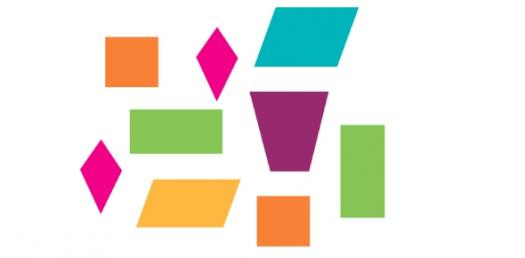10 Questions | Total Attempts: 618SettingsWhat are the attributes polygons? What are the concepts of polygons? Where can I find polygons? Find out how much you have learned about polygons.

• 1.
In your own words provide five reasons that explain why a right triangle is a polygon.
• 2.
Which of these shapes are not polygons?
• A.

Hexagon

• B.

Parallelogram

• C.

• D.

Sphere

• E.

All of the above

• 3.
A polygon is regular when all sides are...
• A.

Straight

• B.

Straight and Curved

• C.

Curved

• D.

Inward

• E.

All the same length

• 4.
Is this statement true or false?  Polygons can be identified in people and objects.
• 5.
Which explanation best describes a polygon?
• A.

They always have straight sides.

• B.

One angle must be 90 degrees.

• C.

The sides are always the same length.

• D.

Two sides must be concave.

• E.

None of the above.

• 6.
Name a regualr polygon with 4 sides.
• 7.
Which is an example of a polygon?
• A.

Kite

• B.

Ball

• C.

Ring

• D.

Bell

• E.

Rock

• 8.
The line segments of a polygon must never do this?
• 9.
All polygons are two dimensional?
• A.

False

• B.

True

• 10.
A shape can not be a polygon if if has two side because it is not a _________figure.
Related TopicsBack to top